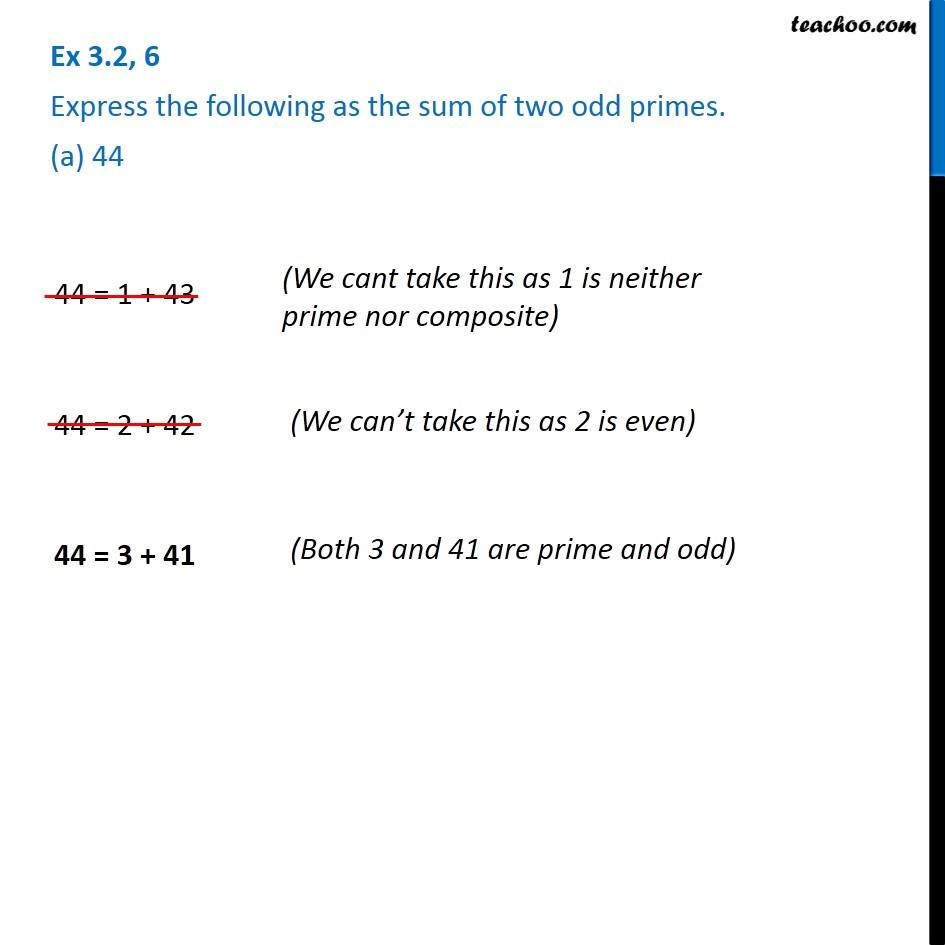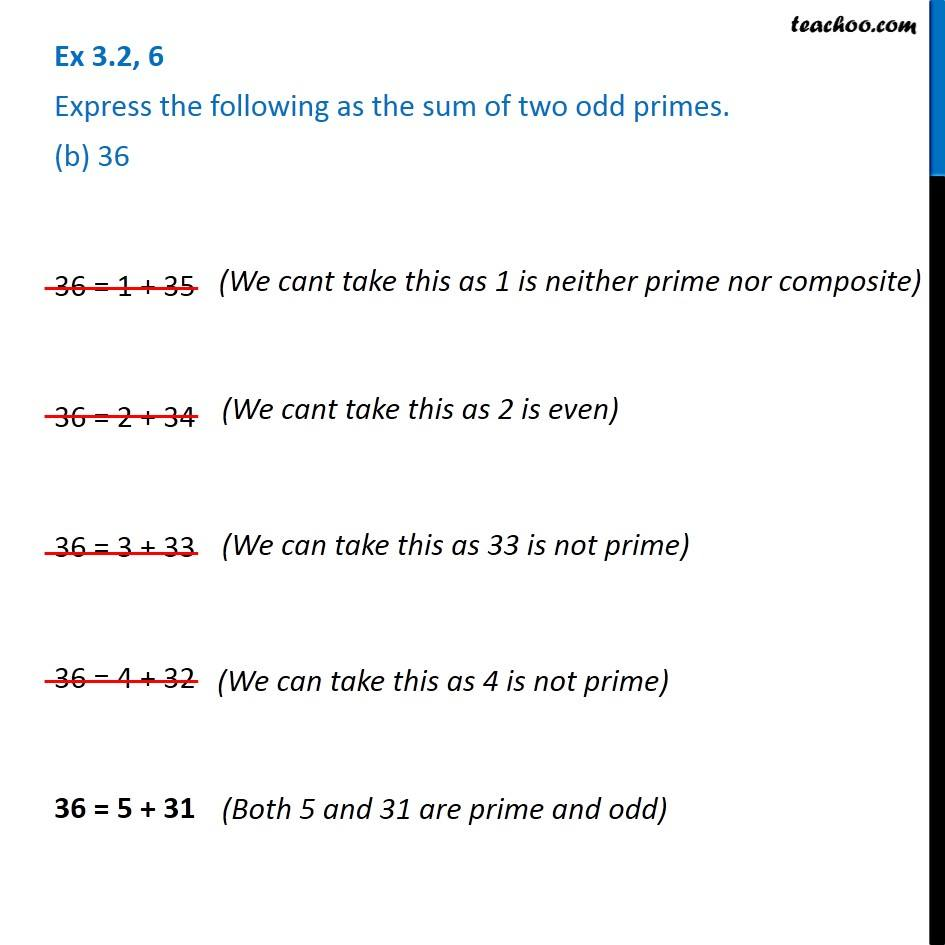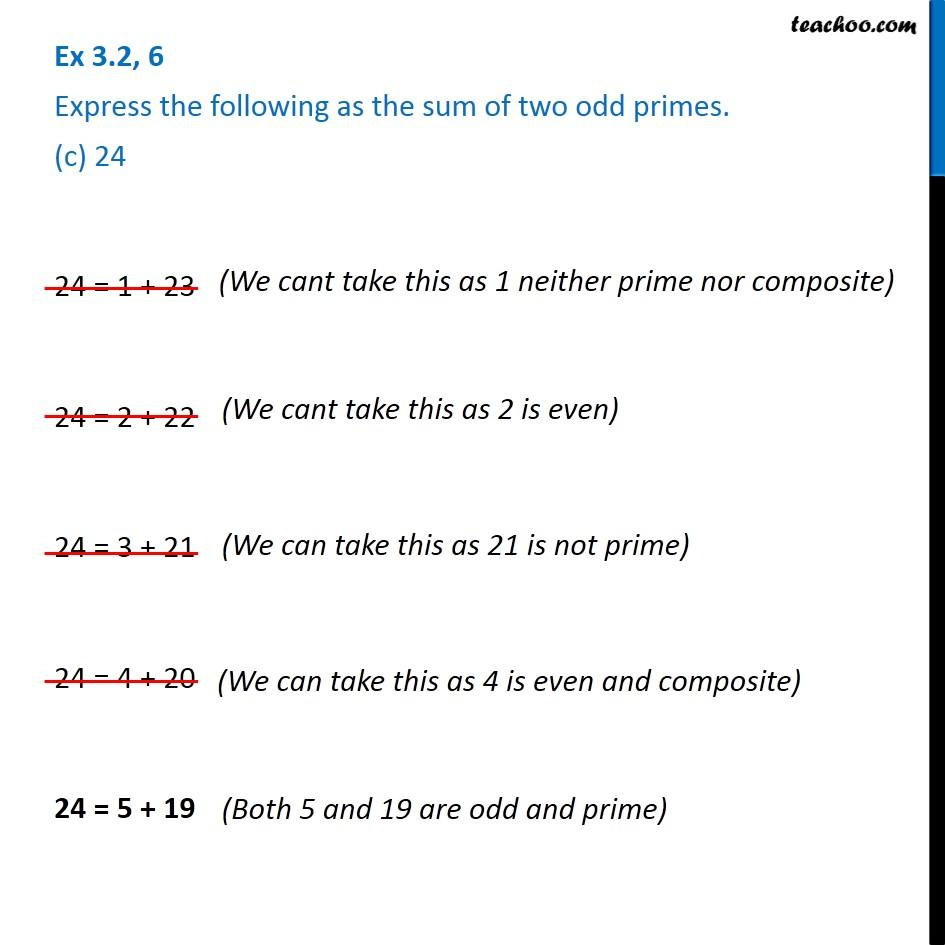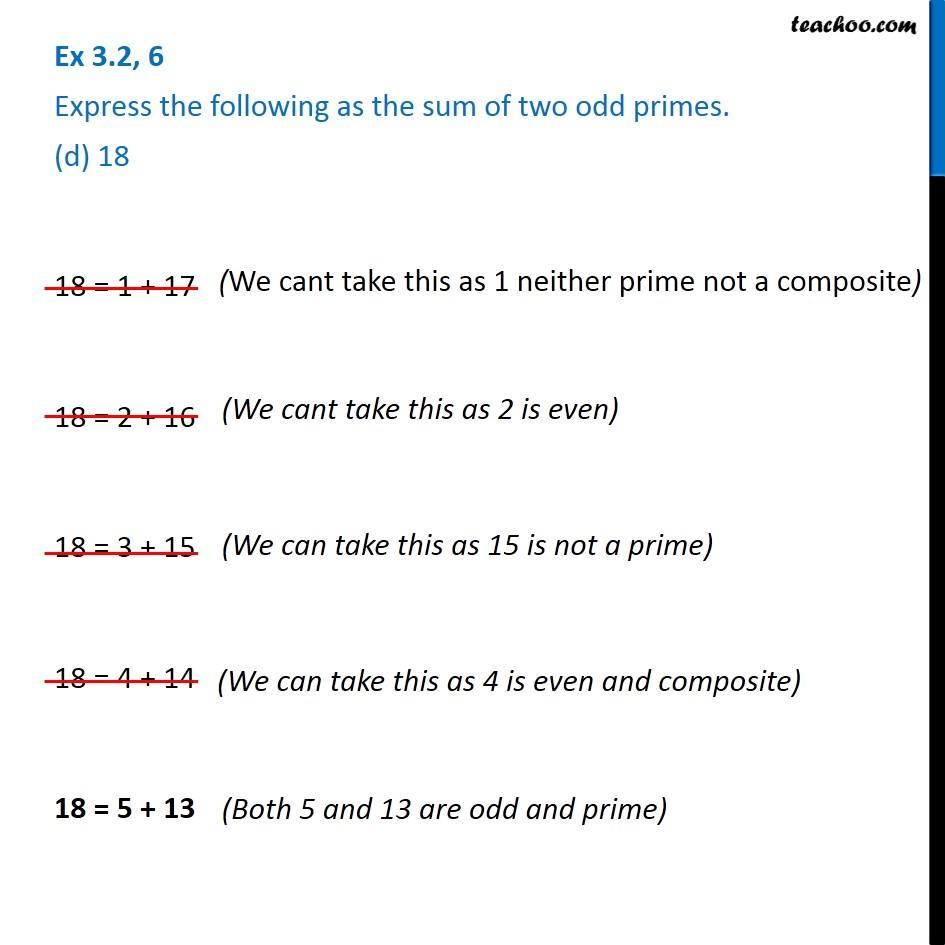Subscribe to our Youtube Channel - https://you.tube/teachoo

1. Chapter 3 Class 6 Playing with Numbers
2. Concept wise
3. Sum of prime numbers

Transcript

Ex 3.2, 6 Express the following as the sum of two odd primes. (a) 4444 = 1 + 43 44 = 2 + 42 44 = 3 + 41 (We cant take this as 1 is neither prime nor composite) (We can’t take this as 2 is even) (Both 3 and 41 are prime and odd) Ex 3.2, 6 Express the following as the sum of two odd primes. (b) 36(We cant take this as 1 is neither prime nor composite) (We cant take this as 2 is even) (We can take this as 33 is not prime) (We can take this as 4 is not prime) Ex 3.2, 6 Express the following as the sum of two odd primes. (c) 24(We cant take this as 1 neither prime nor composite) (We cant take this as 2 is even) 24 = 1 + 23 24 = 2 + 22 24 = 3 + 21 24 = 4 + 20 24 = 5 + 19 (We can take this as 4 is even and composite) (Both 5 and 19 are odd and prime)

Sum of prime numbers